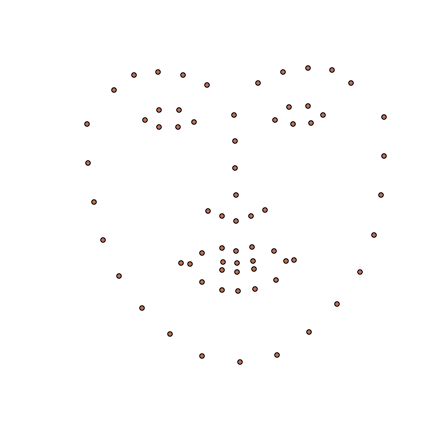In :
%matplotlib inline
import numpy as np


## i·BUG group tutorial

### Joan Alabort-i-Medina

ja310@imperial.ac.uk

## ## Part 1: Building Active Appearance Models

"Active Apperance Models (AAMs) are non-linear, generative, and parametric models of a certain visual phenomenon".

I. Matthews and S. Baker, 2004.
• generative: they generate images of a particular object class (e.g. the human face).

• parametric: they are controlled by a set of parameters.

• non-linear: they are non-linear in terms of pixel intensities.

In :
from menpofit.visualize import visualize_aam

visualize_aam(aam)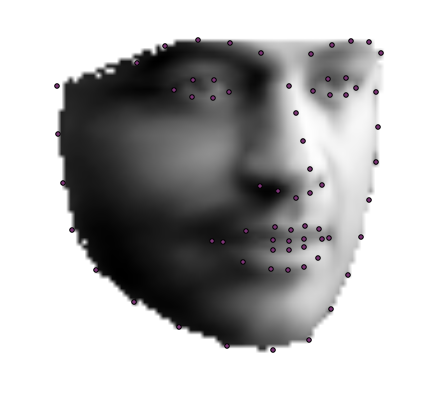A bit of history...

They were originally proposed in 1998 by G. Edwards, C. J. Taylor, and T. F. Cootes from Department of Medical Biophysics (not Computing!) at University of Manchester.

• G. Edwards, C. J. Taylor, and T. F. Cootes, "Interpreting face images using active appearance models". FG 1998.

Luckily for the original authors quite a lot of research stemmed from the original paper:

• T. F. Cootes, K. Walker, and C. J. Taylor, “View-based active appearance models,” in FG, 2000
• T. F. Cootes, G. J. Edwards, and C. J. Taylor, “Active appearance models,” TPAMI, 2001.
• T. F. Cootes and C. J. Taylor, “On representing edge structure for model matching,” in CVPR, 2001
• I. Matthews and S. Baker, “Active appearance models revisited,” IJCV, 2004.
• R. Gross, I. Matthews, and S. Baker, “Generic vs. person specific active appearance models,” IVC, 2005.
• A. U. Batur and M. H. Hayes, “Adaptive active appearance models,” TIP, 2005.
• G. Papandreou and P. Maragos, “Adaptive and constrained algorithms for inverse compositional active appearance model fitting,” CVPR, 2008.
• J. Saragih and R. Gocke, “Learning aam fitting through simulation,” PR, 2009.
• B. Amberg, A. Blake, and T. Vetter, “On compositional image alignment, with an application to active appearance models,” CVPR, 2009.
• G. Tzimiropoulos, J. Alabort-i-Medina, S. Zafeiriou, and M. Pantic. "Generic active appearance models revisited". ACCV, 2012.
• G. Tzimiropoulos and M. Pantic. "Optimization problems for fast aam fitting in-the-wild". ICCV, 2013.
• G. Tzimiropoulos and M. Pantic. "Gauss-Newton deformable part models for face alignment in-the-wild". CVPR, 2014.
• J. Alabort-i-Medina and S. Zafeiriou. "Bayesian active appearance models". CVPR, 2014.
• E. Antonakos, J. Alabort-i-Medina, G. Tzimiropoulos, and S. Zafeiriou. "HOG active appearance models". ICIP 2014.
• J. Kossaifi, G. Tzimiropoulos, M. Pantic. "Fast newton active appearance models". ICIP, 2014.
• J. Alabort-i-Medina, E. Antonakos, J. Booth, P. Snape, S. Zafeiriou. "Menpo: A Comprehensive Platform for Parametric Image Alignment and Visual Deformable Models". ACM Multimedia, Open Source Software Competition, 2014.

although, (typically) linear in terms of both shape and texture, the image formation process is non-linear in terms of pixel intensities

To get us started with AAMs, we will assume that the object we are interested in modelling is no other than the human face:Alt text

The first thing we will need is a large collection of face images:

In :
import menpo.io as mio
from menpo.landmark import labeller, ibug_face_66

images = []
for i  in mio.import_images('/Users/joan/PhD/DataBases/faces/lfpw/trainset/',
max_images=None, verbose=True):

i.crop_to_landmarks_proportion_inplace(0.5)
i = i.rescale_landmarks_to_diagonal_range(100)
labeller(i, 'PTS', ibug_face_66)
if i.n_channels == 3:
i = i.as_greyscale(mode='luminosity')

images.append(i)

- Loading 811 assets: [====================] 100%

In :
from menpo.visualize import visualize_images

visualize_images(images)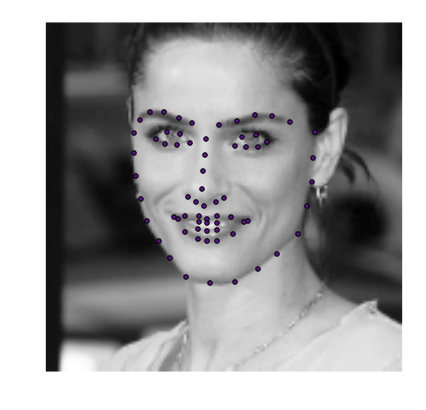Wait a moment... What are these points!

Fair enough, I kind of lied before...

What we really need is a large collection of carefully * annotated* face images.

The previous annotations try to encode the notion face shape...

A shape is the form of an object or its external boundary, outline, or external surface, as opposed to other properties such as colour, texture or material composition.

Wikipedia

...by consistently identifying the positions of a small set of landmarks defining the faces in all images.

In morphometrics, landmark point or shortly landmark is a point in a shape object in which correspondences between and within the populations of the object are preserved.

Wikipedia

Mathematically, a shape can be defined as:

s=(x1,y1,,xv,yv)TR2v×1

In :
from menpo.visualize import visualize_shapes

visualize_shapes([i.landmarks for i in images])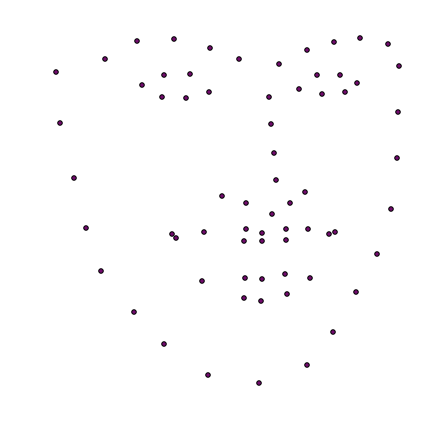In AAMs, images of a particular object are generated by combining linear models describing the shape and texture of the object using a specific motion model (also referred to as the warp).

Let us start by formally defining the shape model:

s=s¯+i=1nspisi=s¯+Sp

where:

s¯=(x1¯,y1¯,,xv¯,yv¯)TR2v

p=(p1,,pns)TRns

S=(s1,,sns)R2v×ns

The previous shape model can be learned by applying Principal Component Analysis (PCA) to the set of manually annotated points defining the object shape in the images (usually after registering all shapes using Procrustes Analysis).

In :
from menpo.transform import Translation, GeneralizedProcrustesAnalysis
from menpo.model import PCAModel

# extract shapes from images
shapes = [i.landmarks['ibug_face_66'].lms for i in images]

# centralize shapes
centered_shapes = [Translation(-s.centre()).apply(s) for s in shapes]
# align centralized shape using Procrustes Analysis
gpa = GeneralizedProcrustesAnalysis(centered_shapes)
aligned_shapes = [s.aligned_source() for s in gpa.transforms]

# build shape model
shape_model = PCAModel(aligned_shapes)

In :
from menpofit.visualize import visualize_shape_model

visualize_shape_model(shape_model)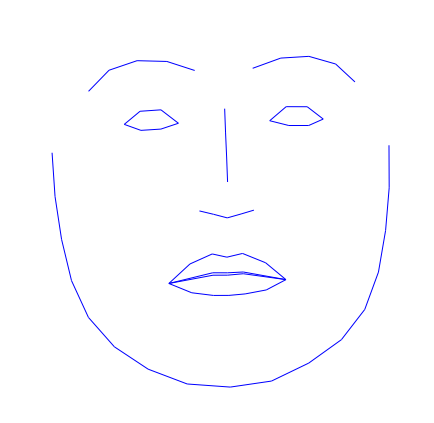Note that because the shapes were normalized using Procrustes Analysis before we applied PCA, the previous shape model has mainly learned nonrigid facial deformations and lacks the ability of placing shapes at arbitrary positions on the image plane.

Luckly, this problem can be solved by composing the model with a 2d similarity transform:

x=sR(x¯+i=1nspixi)+t

where:

x=(x,y)TR2

sR

RR2×2

t=(tx,ty)TR2

Luckily after some clever reparameterization (Matthews and Baker, 2004) the shape model can still be concisely expressed as before:

s=s¯+i=14pisi+i=1nspisi=s¯+Sp+Sp=s¯+S~p~

where:

p=(p1,,p4)TR4

S=(s1,,s4)TR2v×4

s1=s¯R2v

s2=(y1,x1,,yv,xv)TR2v

s3=(1,0,,1,0)TR2v

s4=(0,1,,0,1)TR2v

In :
import numpy as np
from menpo.transform import AlignmentSimilarity
from menpo.model import MeanInstanceLinearModel
from menpofit.modelinstance import OrthoPDM

# get shape model mean as numpy array
shape_vector = shape_model.mean().as_vector()

# initialize S star
S_star = np.zeros((4, shape_vector.shape))
# first column is the mean
S_star[0, :] = shape_vector  # Comp. 1 - just the mean
# second column is the rotated mean
rotated_ccw = shape_model.mean().points[:, ::-1].copy()  # flip x,y -> y,x
rotated_ccw[:, 0] = -rotated_ccw[:, 0]  # negate (old) y
S_star[1, :] = rotated_ccw.flatten()  # C2 - the mean rotated 90 degs
# third column
S_star[2, ::2] = 1  # Tx
# fourth column
S_star[3, 1::2] = 1  # Ty

# build 2d similarity model
sim_2d_model = MeanInstanceLinearModel(S_star, shape_vector, shape_model.mean())

# orthogonalize and compose 2d similarity model with original shape model
augmented_sm = shape_model.copy()
augmented_sm.orthonormalize_against_inplace(sim_2d_model)

In :
visualize_shape_model(augmented_sm)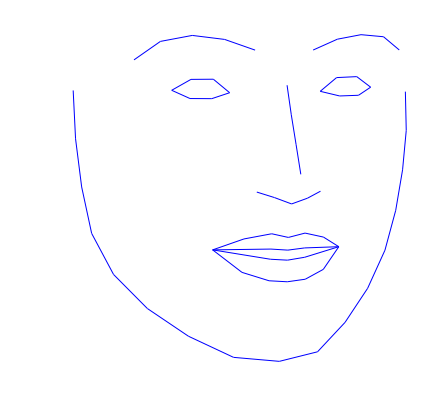In :
from menpofit.transform import DifferentiableAlignmentSimilarity
from menpofit.modelinstance import OrthoPDM

augmented_sm = OrthoPDM(shape_model, AlignmentSimilarity)

In :
visualize_shape_model(augmented_sm.model)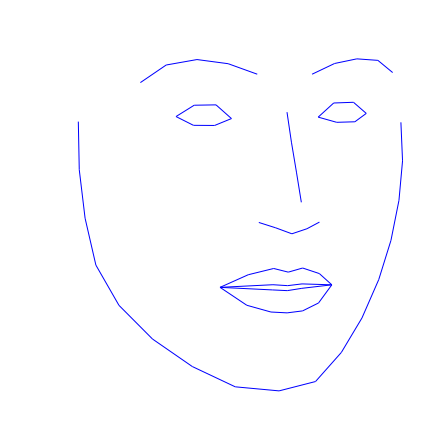So far, so good ;-)

Apart from that las bit...

Let us now switch our attention to the appearance model.

We will shortly see how the appearance model is also learned using PCA. However, in order to be able to apply PCA we first need to introduce the motion model and the concept of shape-free textures.

PCA can only be applied in a particular vector space or, in other words, all vectors to which we want to apply PCA to must have the same lenght.

This is clearly not the case for the face images we just loaded:

In :
print 'image 0 is:', images
print 'image 1 is:', images

image 0 is: 142W x 135H 2D Image with 1 channel
image 1 is: 141W x 140H 2D Image with 1 channel



We could resize all images to a particular resolution but that is very likely to arbitrarily modify their original aspect ratio and include a lot of backgorund information (even if images were tighly cropped).

Instead, the idea is to make use of the annotated landmarks (which are a requirement) to define the face appearance region in each image and map it to the same vector space.

This can be done by:

• Defining a reference image frame (typically build using the shape model mean shape).
• Warping all images to the previous reference frame by using a non-linear warping function.

The non-linear warping function is referred to as the motion model and typical choices for this function include Piece Wise Affine and Thin Plate Splines warps.

Once all images have been warped onto the reference frame they all have the same dimensionality (i.e. they all have the same number of pixels) and we are ready to apply PCA.

Note that, after they have being warped, all images also share the same face shape and hence the name shape-free textures.

A shape free texture can be mathematically defined using the following expression:

vec(I(W(x;p)))=a

In :
from menpo.transform import PiecewiseAffine
from menpofit.aam.builder import build_reference_frame

# build reference frame
reference_frame = build_reference_frame(shape_model.mean())
reference_shape = reference_frame.landmarks['source'].lms

# build PiecewiseAffine transforms
transforms = [PiecewiseAffine(reference_shape, s) for s in shapes]

# warp images
warped_images = []
for (i, t) in zip(images, transforms):
wi.landmarks = reference_frame.landmarks
warped_images.append(wi)

In :
visualize_images(warped_images)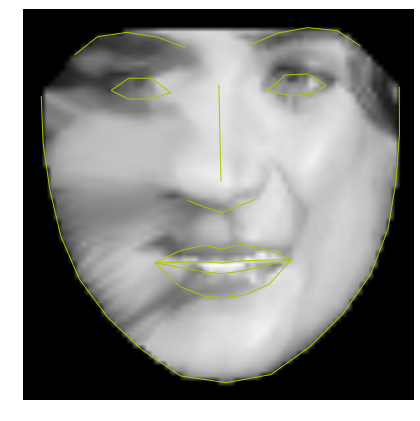After defining the motion model and introducing the concept of shape-free textures, we are now ready to formally define the appearance model:

a=a¯+i=1naciai=a¯+Ac

where:

c=(c1,,cnt)TRnt

A=(a1,,ans)Rd×nt

In :
appearance_model = PCAModel(warped_images)

In :
from menpofit.visualize import visualize_appearance_model

visualize_appearance_model(appearance_model)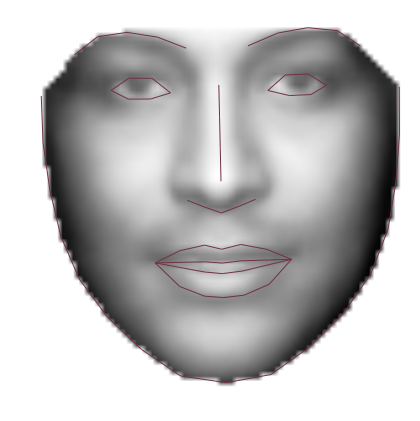Well done!!! We have now almost covered the basics concepts defining Active Appearance Models.

Only one bit is missing, and that is how to combine the previous three models (shape, appearance and motion) so that we can effectively generate novel face images using AAMs.

• Generate a novel shape instance:

s=s¯+Sp

• Generate a novel appearance instance and rearrange it back onto a shape-free texture form:

a=a¯+Ac

A(x)=matrix(a)

• Warp the shape-free appearance intance onto the shape instance using the motion model:

A(W(x,p))=I(x)

In :
# choose shape parameters at random
p = (np.random.randn(shape_model.n_components) *
np.sqrt(shape_model.eigenvalues))
# generate shape instance
s = shape_model.instance(p)

# define image frame containing shape instance
I = build_reference_frame(s)
landmarks = I.landmarks['source'].lms

In :
I.view_landmarks(group='source', render_numbering=False)

Out:
<menpo.visualize.viewmatplotlib.MatplotlibLandmarkViewer2d at 0x11d374c50>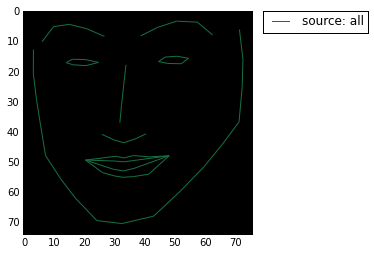In :
 # choose appearance parameters at random
c = (np.random.randn(appearance_model.n_components) *
np.sqrt(appearance_model.eigenvalues))
# generate shape instance
A = appearance_model.instance(c)

In :
A.view()

Out:
<menpo.visualize.viewmatplotlib.MatplotlibImageViewer2d at 0x11e405ed0>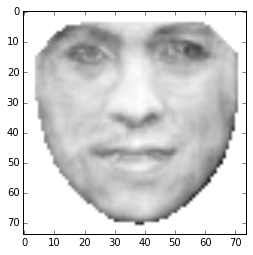In :
# compute PiecewiseAffine transform
transform = PiecewiseAffine(landmarks, A.landmarks['source'].lms)


In :
I.view_landmarks(group='source', render_numbering=False)

Out:
<menpo.visualize.viewmatplotlib.MatplotlibLandmarkViewer2d at 0x11b4d55d0>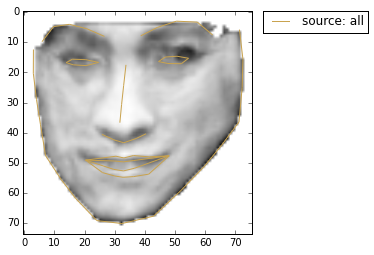Things to take with you from this first part:

• AAMs are non-linear, generative, and parametric models of visual phenomena.

• They consists of three different sub-models:
• Shape model
• Appearance model
• Motion model

• Shape and appearance models are linear models learned from annotated training data using PCA.

• The motion model non-linearly relates the shape and appearance models and is itself an essential part of the AAM formulation.

## ## Part 2: Fitting Active Appearance Models

AAMs were originally developed for solving non-rigid object alignment problems. And up until now they remain quite popular in the domains of face alignment and medical image registration.

As we did in part one, we will find it useful to restrict the problem to the domain faces, i.e. we will use AAMs to specifically tackle the face alignment problem.

Let us start by defining the problem:

Fitting an Active Appearance Model consists of finding the optimal parameters for which its shape and appearance models accurately describe the object being modelled in a particular image.

This definition is mine! :-)

Note that the only available information at fitting time is:

• The input image which we want to fit.
In :
# load image
img = mio.import_image('/Users/joan/PhD/DataBases/faces/lfpw/testset/image_0001.png')

# pre-processing
img.crop_to_landmarks_proportion_inplace(0.5)
img = img.rescale_landmarks_to_diagonal_range(100)
labeller(img, 'PTS', ibug_face_66)
if img.n_channels == 3:
img = img.as_greyscale(mode='luminosity')

In :
img.view()

Out:
<menpo.visualize.viewmatplotlib.MatplotlibImageViewer2d at 0x10c480510>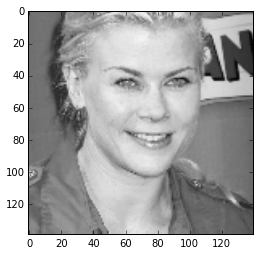• An initial guess for the face shape.
In :
from menpofit.base import noisy_align

# noisy aligned the shape model's mean with the ground truth
transform = noisy_align(shape_model.mean(),
img.landmarks['ibug_face_66'].lms)
initial_shape = transform.apply(shape_model.mean())

# add the initial shape as landmarks to the image
img.landmarks['initial_guess'] = initial_shape

In :
img.view_landmarks(group='initial_guess', render_numbering=False);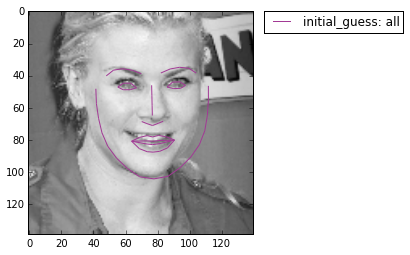• The AAM that we will use to fit the image.
In :
from alabortijcv2015.utils import pickle_load
from menpofit.visualize import visualize_aam